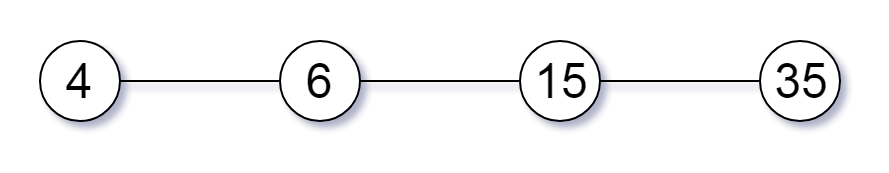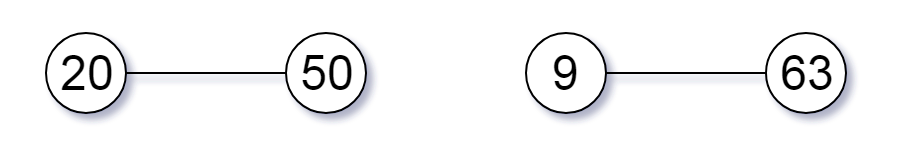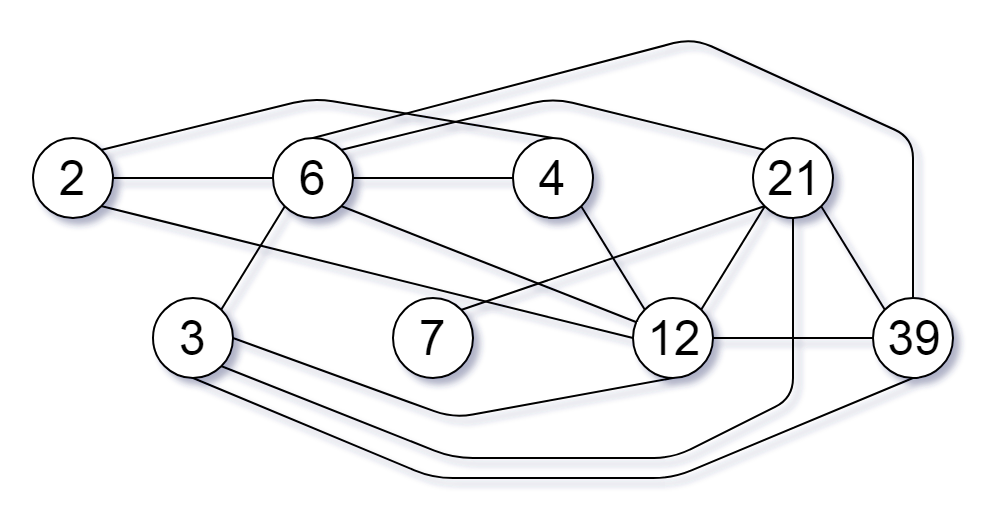0%

### 题目描述

Given a non-empty array of unique positive integers A, consider the following graph:

• There are A.length nodes, labelled A to A[A.length - 1];
• There is an edge between A[i] and A[j] if and only if A[i] and A[j] share a common factor greater than 1.
Return the size of the largest connected component in the graph.

### 例子

#### 例子 1

Input: [4,6,15,35]
Output: 4
Explanation#### 例子 2

Input: [20,50,9,63]
Output: 2
Explanation#### 例子 3

Input: [2,3,6,7,4,12,21,39]
Output: 8
Explanation### Note

1. `1 <= A.length <= 20000`
2. `1 <= A[i] <= 100000`

### 解题思路

• 时间复杂度: O(n * sqrt(M))
• 空间复杂度: O(M)